# Geodesic in a metric space

Jump to: navigation, search

## Definition

### Given data

Let$M$ be a metric space with metric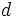$d$. Then, a curve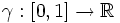$\gamma:[0,1] \to \R$ (or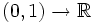$(0,1) \to \R$ or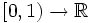$[0,1) \to \R$) is termed a geodesic if for any$a \in [0,1]$, there exists an open subset$U$ of$a$ in$[0,1]$ such that the restriction of$\gamma$ to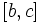$[b,c]$ is the shortest path between them in the metric space, for any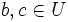$b,c \in U$.

If it is true that the geodesic is the shortest path between its endpoints, it is termed a minimizing geodesic.